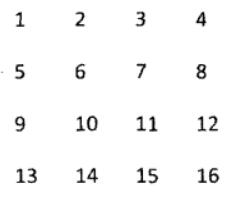# 剑指Offer（十九）：顺时针打印矩阵

csdn：https://blog.csdn.net/baidu_31657889/
github：https://github.com/aimi-cn/AILearners

## 二、题目

### 1、思路1 2 3
4 5 6
7 8 9

6 9
5 8
4 7

### 2、编程实现

python2.7

# -*- coding:utf-8 -*-
class Solution:
# matrix类型为二维列表，需要返回列表
def printMatrix(self, matrix):
# write code here
result = []
while(matrix):
# list.pop详细介绍请看：https://www.runoob.com/python/att-list-pop.html
result+=matrix.pop(0)
if not matrix or not matrix:
break
matrix = self.turn(matrix)
return result
def turn(self,matrix):
num_r = len(matrix)
num_c = len(matrix)
newmat = []
for i in range(num_c):
newmat2 = []
for j in range(num_r):
newmat2.append(matrix[j][i])
newmat.append(newmat2)
newmat.reverse()
return newmat

AIMI-CN AI学习交流群【1015286623】 获取更多AI资料